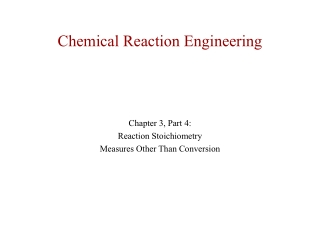DownloadDownload PresentationChemical Reaction Engineering

# Chemical Reaction Engineering

Download Presentation## Chemical Reaction Engineering

- - - - - - - - - - - - - - - - - - - - - - - - - - - E N D - - - - - - - - - - - - - - - - - - - - - - - - - - -
##### Presentation Transcript

1. Chemical Reaction Engineering Chapter 3, Part 4: Reaction Stoichiometry Measures Other Than Conversion

2. Measures Other Than Conversion Uses: A. Membrane reactors B. Multiple reaction Liquids: Use concentrations, i.e., CA

3. Measures Other Than Conversion Uses: A. Membrane reactors • B. Multiple reaction • Liquids: Use concentrations, i.e., CA • For the elementary liquid phase reaction carried out in a CSTR, where V, vo,CAo,k, and Kc are given and the feed is pure A, the combined mole balance, rate laws, and stoichiometry are:

4. Measures Other Than Conversion Uses: A. Membrane reactors • B. Multiple reaction Liquids: Use concentrations, i.e., CA • For the elementary liquid phase reaction carried out in a CSTR, where V, vo,CAo,k, and Kc are given and the feed is pure A, the combined mole balance, rate laws, and stoichiometry are:

5. Measures Other Than Conversion Uses: A. Membrane reactors • B. Multiple reaction Liquids: Use concentrations, i.e., CA • For the elementary liquid phase reaction carried out in a CSTR, where V, vo,CAo,k, and Kc are given and the feed is pure A, the combined mole balance, rate laws, and stoichiometry are:

6. Measures Other Than Conversion Uses: A. Membrane reactors • B. Multiple reaction Liquids: Use concentrations, i.e., CA • For the elementary liquid phase reaction carried out in a CSTR, where V, vo,CAo,k, and Kc are given and the feed is pure A, the combined mole balance, rate laws, and stoichiometry are:

7. Measures Other Than Conversion

8. Measures Other Than Conversion

9. Measures Other Than Conversion 2. There are two equations, two unknowns, CA and CB

10. Measures Other Than Conversion • Gases: Use molar flow rates, i.e., FI

11. Measures Other Than Conversion • Gases: Use molar flow rates, i.e., FI

12. Measures Other Than Conversion • Gases: Use molar flow rates, i.e., FI

13. Measures Other Than Conversion • Gases: Use molar flow rates, i.e., FI

14. Measures Other Than Conversion • Gases: Use molar flow rates, i.e., FI If the above reaction, ,carried out in the gas phase in a PFR, where V, vo,CAo,k, and Kc are given and the feed is pure A, the combined mole balance, rate laws, and stoichiometry yield, for isothermal operation (T=To) and no pressure drop (DP=0) are:

15. Measures Other Than Conversion • Gases: Use molar flow rates, i.e., FI If the above reaction, ,carried out in the gas phase in a PFR, where V, vo,CAo,k, and Kc are given and the feed is pure A, the combined mole balance, rate laws, and stoichiometry yield, for isothermal operation (T=To) and no pressure drop (DP=0) are:

16. Measures Other Than Conversion • Gases: Use molar flow rates, i.e., FI If the above reaction, ,carried out in the gas phase in a PFR, where V, vo,CAo,k, and Kc are given and the feed is pure A, the combined mole balance, rate laws, and stoichiometry yield, for isothermal operation (T=To) and no pressure drop (DP=0) are: Use Polymath to plot FA and FB down the length of the reactor.

17. Measures Other Than Conversion Given: Use Polymath to plot FA and FB down the length of the reactor.

18. Measures Other Than Conversion Given: Use Polymath to plot FA and FB down the length of the reactor. FA FB V

19. Summary At the start of the chapter we saw we needed -rA=f(X). This result is achieved in two steps.

20. Summary At the start of the chapter we saw we needed -rA=f(X). This result is achieved in two steps. • Rate Laws • -rA=k f(Ci) • 1st order A--> B or 1st order -rA=kCA

21. Summary At the start of the chapter we saw we needed -rA=f(X). This result is achieved in two steps. • Rate Laws • -rA=k f(Ci) • 1st order A--> B or 1st order • 2nd order A+B --> C • Rate laws are found by experiment -rA=kCA -rA=kACACB

22. Summary At the start of the chapter we saw we needed -rA=f(X). This result is achieved in two steps. • Rate Laws • -rA=k f(Ci) • 1st order A--> B or 1st order • 2nd order A+B --> C • Rate laws are found by experiment • Stoichiometry • Liquid: • Gas: -rA=kCA -rA=kACACB